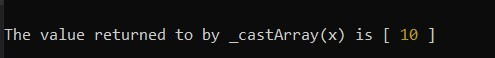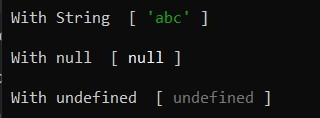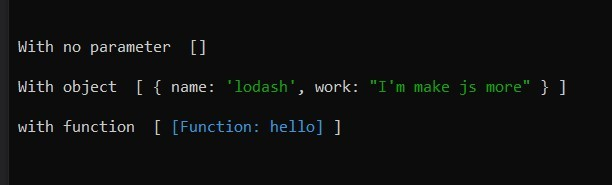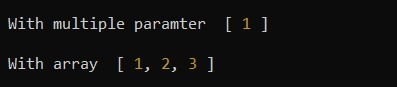# Lodash _.castArray() Method

The _.castArray() method is used to cast value into an array if it is not an array.

Syntax:

`_.castArray( value )`

Parameters: This method accepts single parameter as mentioned above and described below:

• value: This parameter holds the value that need to be inspect.

Return Value: It returns an array with the including value passed in the _.castArray().

Example 1: This example using integer value as a parameter.

## Javascript

 `const _ = require(``'lodash'``); ` ` `  `let x = 10; ` ` `  `let arr = _.castArray(x); ` ` `  `console.log(``"\n\nThe value returned to by _castArray(x) is"``, arr); `

Here, const _ = require(‘lodash’) is used to import the lodash library into the file.
Output:Example 2: This example using String, null, and undefined as a parameter.

## Javascript

 `const _ = require(``'lodash'``); ` ` `  `let x = _.castArray(``'abc'``); ` ` `  `console.log(``'\n With String '``, x ); ` `  `  `let y = _.castArray(``null``); ` ` `  `console.log(``'\n With null '``, y ); ` `  `  `let z = _.castArray(undefined); ` ` `  `console.log(``'\n With undefined '``, z); `

Output:Example 3: With no parameter, object, and function.

## Javascript

 `const _ = require(``'lodash'``); ` ` `  `let x = _.castArray(); ` ` `  `console.log(``"\n With no parameter "``, x); ` ` `  `let y = _.castArray({``"name"``:``"lodash"``, ` `         ``"work"``:``"I'm make js more"` `}); ` ` `  `console.log(``"\n With object "``, y); ` ` `  `let z = _.castArray(``function` `hello() { ` `    ``console.log(``"hello"``); ` `}); ` ` `  `console.log(``"\n with function "``, z); `

Output:Example 4: This example using multiple parameters, it will take only the first parameter and with an array, it will just return the same array.

## Javascript

 `const _ = require(``'lodash'``); ` ` `  `let x = _.castArray(1, 2, ``"hello"``); ` ` `  `console.log(``'\n With multiple parameter '``, x); ` ` `  `let y = _.castArray([1, 2, 3]); ` ` `  `console.log(``'\n With array '``, y); `

Output:Note: This will not work in normal JavaScript because it requires the library lodash to be installed.
Reference: https://lodash.com/docs/4.17.15#castArray